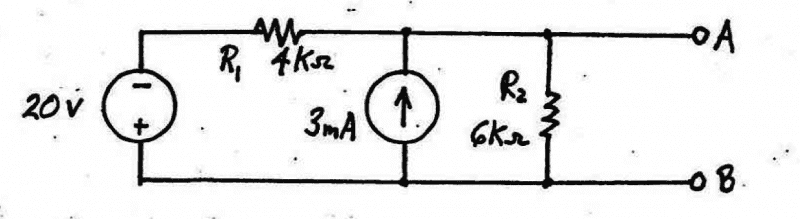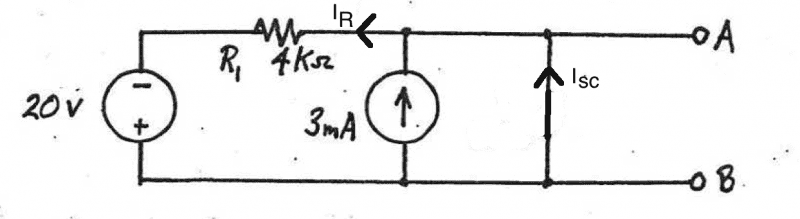# Norton's Theorem and Thevenin's theorem

• STEMucator

Homework Helper

## Homework Statement

For some reason I don't use this theorem since Thevenin's theorem is more common. I'm asked to find the Norton equivalent source between the terminals ##A## and ##B## for the following:## The Attempt at a Solution

The first step is to short ##R_2##, and to find the short circuit current across that wiring.I believe KCL at the node above the ##3 mA## would be useful:

##\sum I = 0 \Rightarrow 3mA + I_{sc} - I_R = 0 \Rightarrow I_{sc} = I_R - 3mA = \frac{V_{Node} + 20V}{4k} - 3mA##

Now ##V_{Node}## is the voltage across the ##3 mA##. Applying KVL around the left loop using the current source as a constraint:

##\sum V = 0 \Rightarrow 20V + V_R + V_{Node} = 0 \Rightarrow V_{Node} = -(20V + V_R) = -(20V + (3mA)(4k)) = - 32V##

This implies that ##I_{sc} = \frac{-32V + 20V}{4k} - 3mA = - 6mA##.

Is this reasonable?

You went astray when you wrote equations with ##V_{Node}##. The short circuit forces ##V_{Node}## to be zero volts. If you think about the Superposition Principle you can take advantage of that, since it makes calculating the current contribution of the 20 V source quite simple.

If I may suggest another approach? Start by converting the 20V source and R1 to their Norton equivalent. Then take advantage of parallel connections to simplify...

•STEMucator
Ah source transforms, those are awfully useful and I hardly ever use them.

First, ##V = -IR \Rightarrow I = \frac{-20V}{4k} = -5mA## so ##-5mA## points up or ##5mA## points down. Also, the ##5 mA## is in parallel with the ##4k## now.

So the combined current in parallel is ##-5mA + 3mA = -2mA##. This is the only current in this new circuit (comprised of the current source, 4k resistor and shorted 6k resistor). Hence ##I_{sc} = -2mA##.

Now going back to the original circuit, short circuiting the voltage and open circuiting the current source, we find:

##R_{th} = (\frac{1}{4k} + \frac{1}{6k})^{-1} = \frac{12k}{5}##

Hence the norton equivalent would be ##-2mA## pointing up in parallel with ##R_{th}##.

There you go! Much less brain sweat required :)